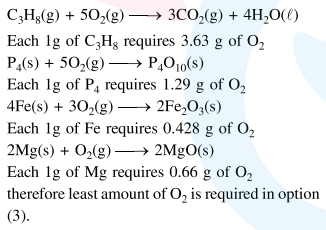Deepak Scored 45->99%ile with Bounce Back Crack Course. You can do it too!

# Solve this following

Question:

The minimum amount of $\mathrm{O}_{2}(\mathrm{~g})$ consumed per gram of reactant is for the reaction:

(Given atomic mass : $\mathrm{Fe}=56, \mathrm{O}=16$, $\mathrm{Mg}=24, \mathrm{P}=31, \mathrm{C}=12, \mathrm{H}=1$ )

1. $\mathrm{C}_{3} \mathrm{H}_{8}(\mathrm{~g})+5 \mathrm{O}_{2}(\mathrm{~g}) \rightarrow 3 \mathrm{CO}_{2}(\mathrm{~g})+4 \mathrm{H}_{2} \mathrm{O}(l)$

2. $\mathrm{P}_{4}(\mathrm{~s})+5 \mathrm{O}_{2}(\mathrm{~g}) \rightarrow \mathrm{P}_{4} \mathrm{O}_{10}(\mathrm{~s})$

3. $4 \mathrm{Fe}(\mathrm{s})+3 \mathrm{O}_{2}(\mathrm{~g}) \rightarrow 2 \mathrm{FeO}_{3}(\mathrm{~s})$

4. $2 \mathrm{Mg}(\mathrm{s})+\mathrm{O}_{2}(\mathrm{~g}) \rightarrow 2 \mathrm{MgO}(\mathrm{s})$

Correct Option: , 3

Solution: